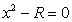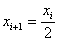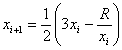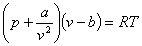Transforming Numerical Methods Education for the STEM Undergraduate MOOC | MOBILE | VIDEOS | BLOG | YOUTUBE | TWITTER | COMMENTS | ANALYTICS | ABOUT | CONTACT | COURSE WEBSITES | BOOKS | MATH FOR COLLEGE
 MULTIPLE CHOICE TEST NEWTON-RAPHSON METHOD NONLINEAR EQUATIONS

Q1. The Newton-Raphson method of finding roots of nonlinear equations falls under the category of _______ methods.
bracketing

open

random

graphical

Q2. The Newton-Raphson method formula for finding the square root of a real number R from the equationis,Q3. The next iterative value of the root ofusing Newton-Raphson method, if the initial guess is 3, is
1.500

2.066

2.167

3.000

Q4. The root of the equation f(x)=0  is found by using the Newton-Raphson method.  The initial estimate of the root is x0=3, and f(3)=5.  The angle the line tangent to the function f(x) makes at x=3 with the x-axis is 570.  The next estimate of the root, x1 most nearly is
-3.247

-0.2470

3.247

6.247

Q5. The root ofis found by using the Newton-Raphson method.  The successive iterative values of the root are given in the table below

 Iteration Number Value of Root 0 2.0000 1 1.6667 2 1.5911 3 1.5874 4 1.5874

The iteration number at which I would first trust at least two significant digits in the answer is

1

2

3

4

Q6. The ideal gas law is given bywhere where p is the pressure, v is the specific volume, R is the universal gas constant, and T is the absolute temperature.  This equation is only accurate for a limited range of pressure and temperature.  Vander Waals came up with an equation that was accurate for larger ranges of pressure and temperature given bywhere a and b are empirical constants dependent on a particular gas.  Given the value of R=0.08, a=3.592, b=0.04267, p=10 and T=300 (assume all units are consistent), one is going to find the specific volume, v, for the above values.  Without finding the solution from the Vander Waals equation, what would be a good initial guess for v?

0
1.2
2.4
4.8

 AUDIENCE |  AWARDS  |  PEOPLE  |  TRACKS  |  DISSEMINATION  |  PUBLICATIONS Copyrights: University of South Florida, 4202 E Fowler Ave, Tampa, FL 33620-5350. All Rights Reserved. Questions, suggestions or comments, contact kaw@eng.usf.edu  This material is based upon work supported by the National Science Foundation under Grant#0126793, 0341468, 0717624,  0836981, , 0836805, 1322586.  Any opinions, findings, and conclusions or recommendations expressed in this material are those of the author(s) and do not necessarily reflect the views of the National Science Foundation.  Other sponsors include Maple, MathCAD, USF, FAMU and MSOE.  Based on a work at http://mathforcollege.com/nm.  Holistic Numerical Methods licensed under a Creative Commons Attribution-NonCommercial-NoDerivs 3.0 Unported License. ANALYTICS